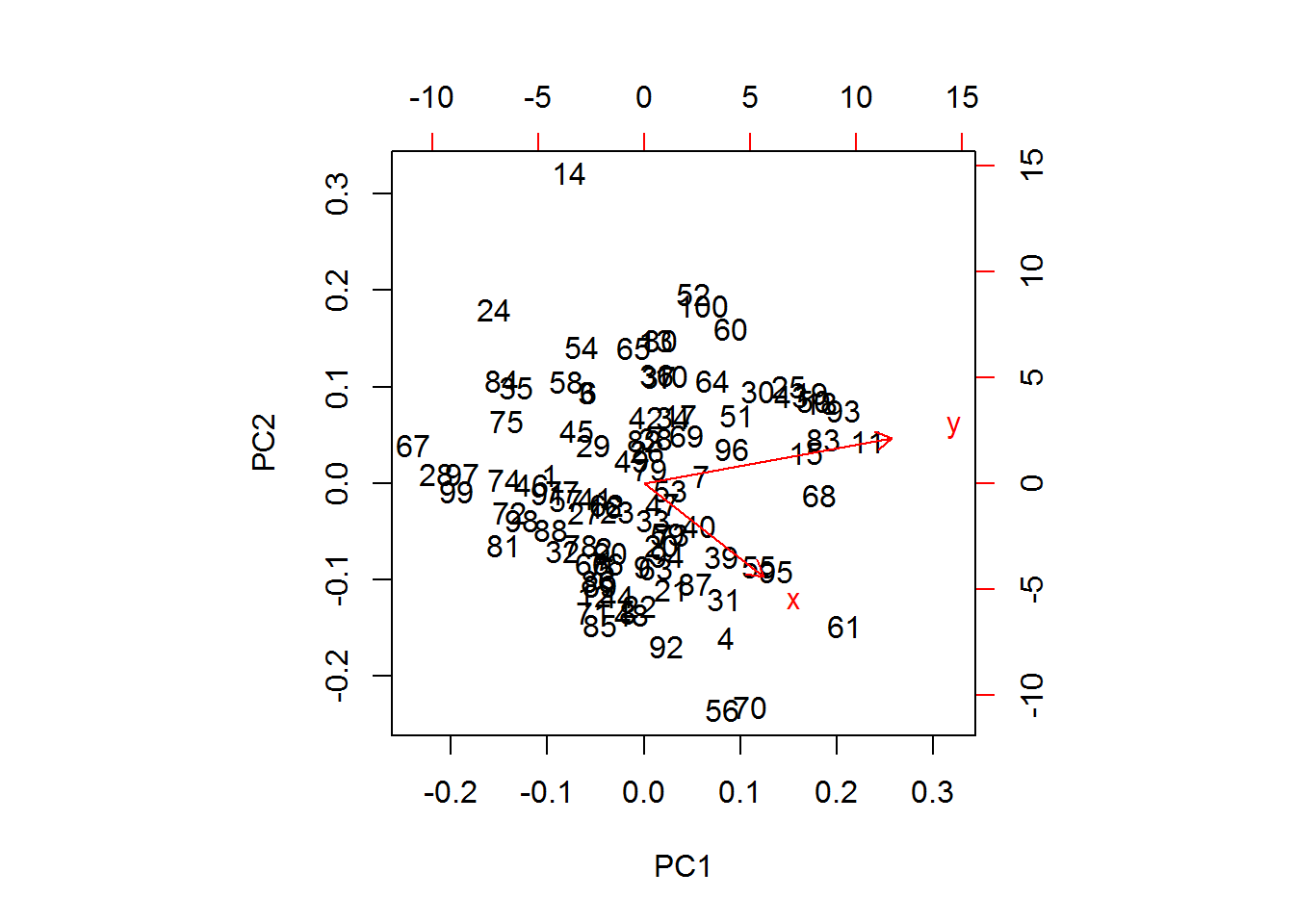• Principal components are the eigenvectors of the data’s covariance matrix

### Eigenvectors

Definition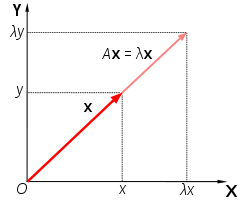• A vector can be multiplied by a matrix to generate a new vector
• “Matrix A maps vector x1 to vector x2”
• For certain vectors, multiplication by a given matrix generates a vector that is a scalar multiple of the original vector
• This makes the original vector an eigenvector of that matrix
• The equivalent constant that would generate the same scalar multiple of the eigenvector is the eigenvalue
• $$A \cdot x = \lambda \cdot x$$
• Where $$A$$ is a matrix, $$x$$ is an eigenvector, and $$\lambda$$ is an eigenvalue
• In the example to the right, $$Ax$$ is a scalar multiple of $$x$$ so $$x$$ is an eigenvector of $$A$$

Graphic interpretation of eigenvectors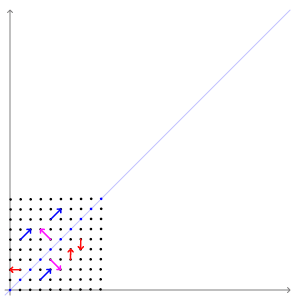• For a set of vectors, multiplying them all by a given matrix results in a transformation of that set
• E.g. if a set of vectors is represented by a 2D grid of points, multiplication by a matrix will transform that grid into a new shape
• An eigenvector is an axis along which that transformation does not change the orientation of any vector
• It is the direction in which the transformation “stretches”
• In the example to the right, the 2D grid is transformed and the arrows represent directions of the various vectors in that space
• Note that while the red vectors change in orientation (up/down or left/right become diagonal), the pink and blue arrows remain in the same orientation
• Thus, the pink and blue arrows are the eigenvectors of this matrix transformation
• Note that the pink and blue arrows are also orthogonal to each other.
• For an $$n x n$$ matrix, there will be $$n$$-number of eigenvectors
• Notice that there are only two eigenvectors shown in the 2D (i.e. 2x2) example to the right
• Observe that the matrix transformation “stretches” the grid and there are only two directions in which a vector could conceivably be unaffected by this stretch
• I.e. The exact direction of the stretch and perpendicular direction (i.e. orthogonal)
• The same principle would apply to a 3x3 (3D) matrix or higher order

Conceptual interpretation of eigenvectors

### Covariance and correlation

Why covariance matrix?

• Any square matrix can serve to transform a set of vectors
• The “directions” of this transformation are represented by the eigenvectors
• Thus, the eigenvectors of a covariance matrix represent the “direction of covariance”
• “Finding the eigenvectors and eigenvalues of the covariance matrix is the equivalent of fitting those straight, principal-component lines to the variance of the data. Why? Because eigenvectors trace the principal lines of force, and the axes of greatest variance and covariance illustrate where the data is most susceptible to change.”

• “Think of it like this: If a variable changes, it is being acted upon by a force known or unknown. If two variables change together, in all likelihood that is either because one is acting upon the other, or they are both subject to the same hidden and unnamed force.”

• “When a matrix performs a linear transformation, eigenvectors trace the lines of force it applies to input; when a matrix is populated with the variance and covariance of the data, eigenvectors reflect the forces that have been applied to the given. One applies force and the other reflects it.”

• “Eigenvalues are simply the coefficients attached to eigenvectors, which give the axes magnitude. In this case, they are the measure of the dataâs covariance. By ranking your eigenvectors in order of their eigenvalues, highest to lowest, you get the principal components in order of significance.”

Calculating covariance

• $$cov(x,y) = \frac{\sum_{i=1}^{n}(x_i-\bar{x})(y_i-\bar{y})}{n-1}$$

df <- iris
#Calculating covariance matrix with built in function
(cv <- cov(df[,-5])) 
##              Sepal.Length Sepal.Width Petal.Length Petal.Width
## Sepal.Length    0.6856935  -0.0424340    1.2743154   0.5162707
## Sepal.Width    -0.0424340   0.1899794   -0.3296564  -0.1216394
## Petal.Length    1.2743154  -0.3296564    3.1162779   1.2956094
## Petal.Width     0.5162707  -0.1216394    1.2956094   0.5810063
#Manually calculate covariance between variables
var1 <- 1; var2 <- 2
sum(apply(apply(df[,var1:var2], 2, function(x) x - mean(x)),1,prod))/(nrow(df)-1) 
##  -0.042434
#R calculated covariance between variables
var1 <- 1; var2 <- 2
cv[var1,var2] #R function
##  -0.042434

Covariance vs correlation (tangent)

• Covariance between different pairs of variable are hard to compare
• Correlation “normalizes” covariance by dividing by each variables standard deviation
• $$cor(x,y) = \frac{\sum_{i=1}^{n}(\frac{x_i-\bar{x}}{\sigma_x})(\frac{y_i-\bar{y}}{\sigma_y})}{n-1} = \frac{cov(x,y)}{\sigma_x\sigma_y}$$
var1 <- 1; var2 <- 2
sum(apply(apply(df[,var1:var2], 2, function(x) (x - mean(x))/sd(x)),1,prod))/(nrow(df)-1)
##  -0.1175698
var1 <- 1; var2 <- 2
cor(df[,-5])[var1,var2]
##  -0.1175698

# Principal components

Calculation of the principal components

• As discussed above, the principal components are the eigenvectors of the covariance matrix

Eigenvalues <- eigen(cv)$values Eigenvectors <- eigen(cv)$vectors
#Manual component loadings
round(Eigenvectors,5)
##          [,1]     [,2]     [,3]     [,4]
## [1,]  0.36139 -0.65659 -0.58203  0.31549
## [2,] -0.08452 -0.73016  0.59791 -0.31972
## [3,]  0.85667  0.17337  0.07624 -0.47984
## [4,]  0.35829  0.07548  0.54583  0.75366
#prcomp component loadings
round(prcomp(df[,-5])$rotation,5) ## PC1 PC2 PC3 PC4 ## Sepal.Length 0.36139 -0.65659 0.58203 0.31549 ## Sepal.Width -0.08452 -0.73016 -0.59791 -0.31972 ## Petal.Length 0.85667 0.17337 -0.07624 -0.47984 ## Petal.Width 0.35829 0.07548 -0.54583 0.75366 Calculation of the PCA data transformation • Transforming the original data by the principal components rotates the data to make the principal components the new axes • This is done by multiplying the raw data by the principal components • PCA-transformed data is often normalized to the mean • However, this will not change the shape of the data, only the scale of the axes #PCA-transformation PC <- as.matrix(df[,-5]) %*% Eigenvectors #Normalization PC <- apply(PC, 2, function(x) x - mean(x)) #Manual PCA transformation head(PC) ## [,1] [,2] [,3] [,4] ## [1,] -2.684126 -0.3193972 -0.02791483 0.002262437 ## [2,] -2.714142 0.1770012 -0.21046427 0.099026550 ## [3,] -2.888991 0.1449494 0.01790026 0.019968390 ## [4,] -2.745343 0.3182990 0.03155937 -0.075575817 ## [5,] -2.728717 -0.3267545 0.09007924 -0.061258593 ## [6,] -2.280860 -0.7413304 0.16867766 -0.024200858 #prcomp PCA transformation head(prcomp(df[,-5])$x)
##            PC1        PC2         PC3          PC4
## [1,] -2.684126 -0.3193972  0.02791483  0.002262437
## [2,] -2.714142  0.1770012  0.21046427  0.099026550
## [3,] -2.888991  0.1449494 -0.01790026  0.019968390
## [4,] -2.745343  0.3182990 -0.03155937 -0.075575817
## [5,] -2.728717 -0.3267545 -0.09007924 -0.061258593
## [6,] -2.280860 -0.7413304 -0.16867766 -0.024200858
• The variances of the resulting transformation should be equivalent to the eigenvalues of the original covariance matrix
• The covariances of the resulting transformation should all be zero
• This is because the prinicpal components are orthogonal and, thus, uncorrelated
round(cov(PC), 3)
##       [,1]  [,2]  [,3]  [,4]
## [1,] 4.228 0.000 0.000 0.000
## [2,] 0.000 0.243 0.000 0.000
## [3,] 0.000 0.000 0.078 0.000
## [4,] 0.000 0.000 0.000 0.024
round(Eigenvalues, 3)
##  4.228 0.243 0.078 0.024

# 2D example

#Generating some random 2D data
library(ggplot2)
set.seed(1)
df.1 <- data.frame("x" = rnorm(100))
df.1$y <- jitter(df.1$x, 1e4) #Adds noise to the points in df$x to create a correlated variable ggplot(df.1, aes(x=x, y=y)) + geom_point() + theme_bw()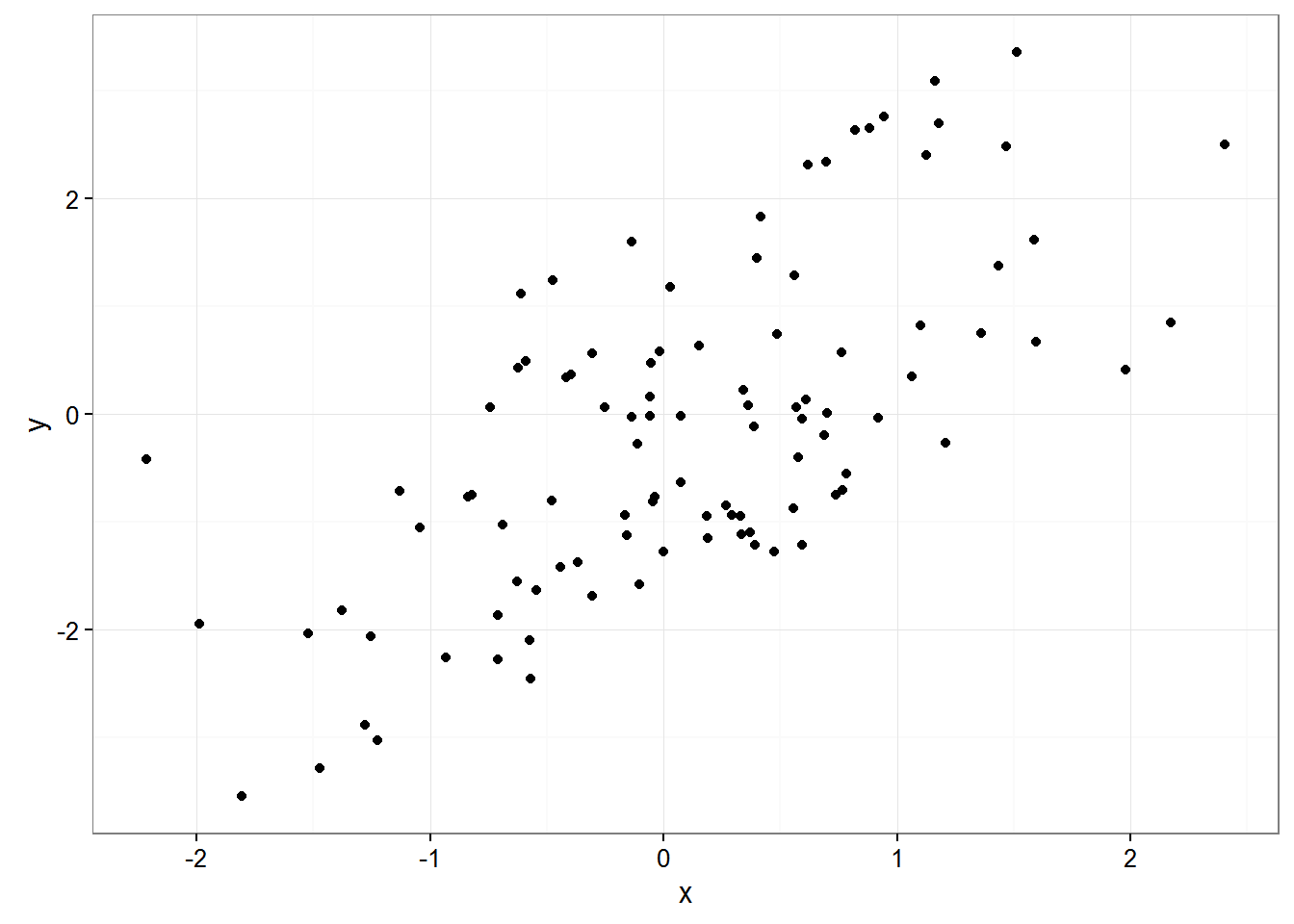cov(df.1) ## x y ## x 0.8067621 0.8901109 ## y 0.8901109 2.2166338 cor(df.1) ## x y ## x 1.000000 0.665617 ## y 0.665617 1.000000 • As can be seen, X and Y correlate well with each other PC.vectors <- eigen(cov(df.1))$vectors
PC1 <- PC.vectors[,1]
PC2 <- PC.vectors[,2]
ggplot(df.1, aes(x=x, y=y)) + theme_bw() +
geom_segment(aes(x = -PC1, y = -PC1, xend = PC1, yend = PC1), color = "red", size = 2) +
geom_segment(aes(x = -PC2, y = -PC2, xend = PC2, yend = PC2), color = "red", size = 2) +
geom_point()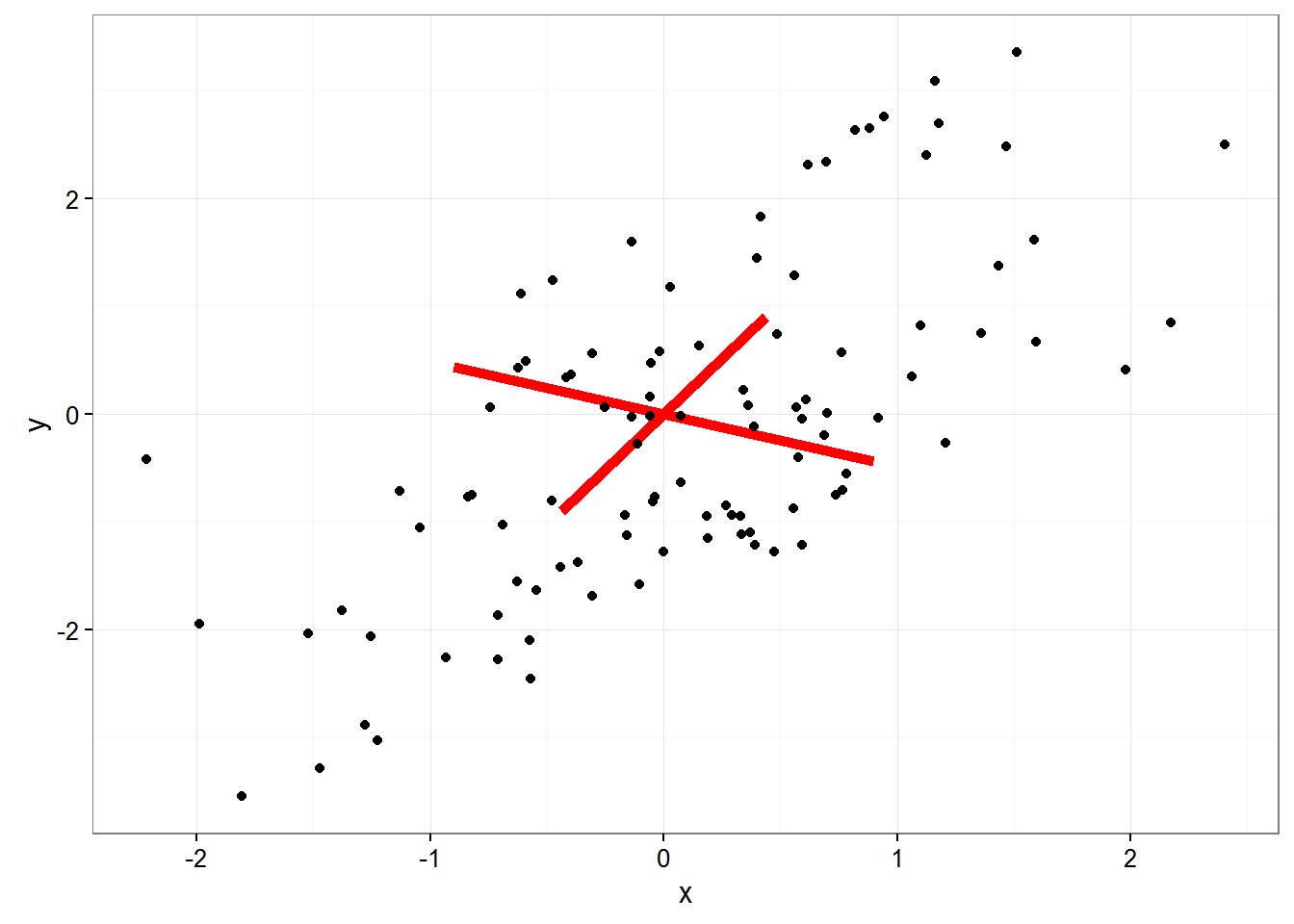• In the above plot, the red lines represent components PC1 and PC2
• PC1 follows the overall correlation of the data
• PC2 is orthogonal to PC1
• May not appear perpendicual in the plot because of scaling, but we can calculate that it is orthogonal
crossprod(PC1, PC2) #The crossproduct of orthogonal vectors should be zero
##      [,1]
## [1,]    0
• Can verify that components PC1 and PC2 are truly orthogonal because their crossproduc is zero
#Manual transformation (par for plot size adjust)
par(pty="s");plot(as.matrix(df.1) %*% PC.vectors)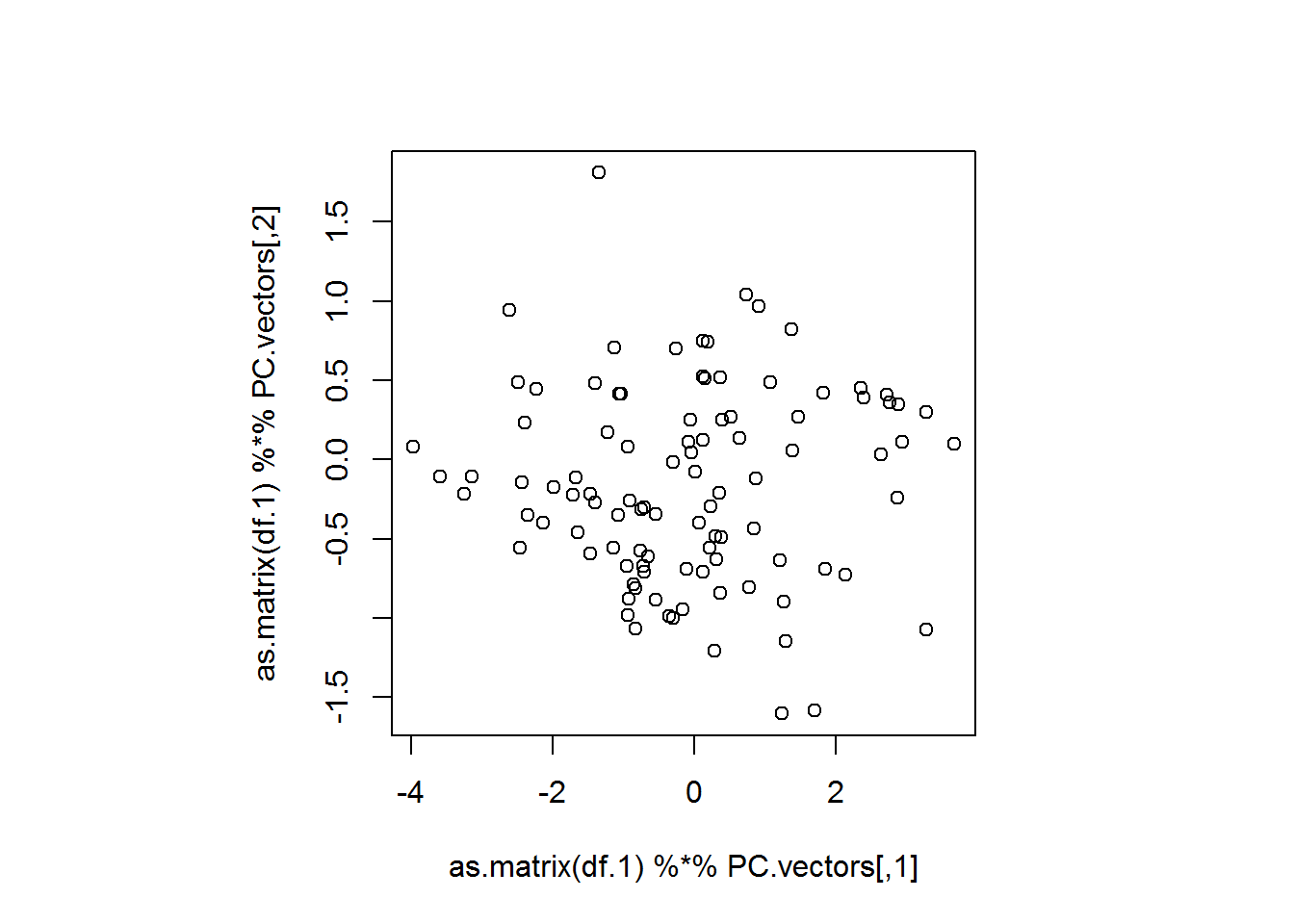#prcomp transformation
biplot(prcomp(df.1))